﻿ The Effect of the Shaft Diameter and Torsional Stiffness on the Whirling Speed of the Ship Propeller Shafting SystemPublications are Open
Access in this journal
Article Versions
Export Article
• Normal Style
• MLA Style
• APA Style
• Chicago Style
Research Article
Open Access Peer-reviewed

### The Effect of the Shaft Diameter and Torsional Stiffness on the Whirling Speed of the Ship Propeller Shafting System

Jabbar Firouzi, Hassan Ghassemi
Journal of Mechanical Design and Vibration. 2017, 5(1), 37-42. DOI: 10.12691/jmdv-5-1-5
Published online: September 27, 2017

### Abstract

Finding the whirling speed of the shaft of the ship propeller is presented on this paper. Power generated from the engine is transmitted to the propeller directly/indirectly using gearbox. This is important to find the natural frequencies of the shafting system in order to prevent resonance. So, multi-DOF (degree of freedom) is considered on this study including engine, gearbox and propeller, flywheel and etc. connecting by the shaft. Whiling speeds of the shaft are determined at various conditions as well as the effect of the shaft torsional stiffness and shaft diameter are presented and discussed.

### 1. Introduction

Generally, the propulsion shaft vibration that can occur on a ship can be divided into three types or modes, which are torsional and axial coupled by torsional and whirling vibration. Usually this whirling vibration in the design stage is not considered.

Recently, the blade arrangement in the whirling has been reduced to almost the operating range of the propulsion shafting system, thus increasing the moment of inertia and the mass of the propeller due to the large and larger vessels. Additionally, the forward stern tube bearing is often removed to some extent in order to maintain the flexibility of shaft alignment, and hence the vertical supporting stiffness decreases. For this reason, the failure of the shaft systems related to whirling increases.

The whirling speeds and mode shapes are important dynamic characteristics of a rotational system, because they are required in the analysis of forced vibration response. The exact prediction of higher whirl speeds is more and more important with increasing demand for design of rotating machines 1.

Suk Sun et al, Studied the measurement and analysis of the whirling vibration behavior of the propulsion shafting system using Gap-sensors in a container vessel. They performed the theory of whirling vibration before measurements and then measured based on the results of the analysis, such as the natural frequency and vibration mode shape. Based on the results, more accurate methods were proposed to assessment the natural frequency of the whirling vibration 2.

Batrak et al presented the results of the free and forced whirling vibration analysis obtained using special software intended to shaft design. They discussed the issues related to the further analysis and development of the whirling vibration calculation of the propulsion shafting system 3.

Ku carried out a finite element model for testing the speed and stability of rotor- bearing systems. In addition to the effects of translational and rotatory inertia and gyroscopic moments, combined effects of transverse shear deformations, internal viscous and hysterical damping weres combined in formulation. In order to evaluate the accuracy of the available finite element model, the natural frequencies of a non-rotating simply supported shaft were first examined the results were highly accurate 4.

Shahgholi et al investigated the effects of inequality between two eccentricities axes on principal axes and external damping on the steady-states and bifurcation points of asymmetric rotating shaft. The numerical calculations were performed to study the results of the harmonic balance method, which results of the harmonic balancing method in accordance with numerical calculations. Also, the initial and parametric resonance of a simply supported nonlinear rotating asymmetrical shaft with unequal mass moments of inertia and bending stiffness in the direction of the principal axis were considered simultaneously. Then they applied multiple scales method on the ordinary and partial differential equations of motion 5, 6.

Tailony presented a lumped mass model of an internal combustion engine for the cold test torsional system to perform high-accuracy driveline natural frequency calculations. Since the natural frequency of the system is necessary to prevent resonance stimulation during the test phase, the Eigen value problem was solved and also obtains the natural frequency and mode shapes 7.

Wu et al provided a method for replacing the effects of each rigid disk that was mounted on the rotating shaft by a lumped mass along with a frequency corresponding to the equivalent mass moment of inertia, so that the whirling motion of a rotating shaft disk system, like a transverse free vibration of a fixed beam In which the free vibration analysis technique of a fixed beam with multiple concentrated elements can be used to determine the forward and backward whirling speeds along with mode shape of a distributed-mass shaft carrying arbitrary rigid disk 8.

Desavale et al performed a complete analysis of a crankshaft for torsional and bending vibrations to identification of stresses with finite element method. They analyzed the natural frequency, rigid body mode shape by ANSYS and Holzer method, and performed a complete simulation of the actual boundary conditions for journal bearing support, inertia lumping for reciprocating parts and bearing stiffness 9.

Zou et al first longitudinal resonance of a marine propulsion shaft system with particular attention to the internal resonance between the longitudinal and transverse directions studied treaty. Then, the longitudinal and transverse coupling dynamical equations were created using the Ritz method and the Lagrange equation, solved by multiple-scale method 10, 11.

Tecnavin presented a review of cases of propulsion systems in which there are noise, vibration or fracture problems. Various vessels were analyzed based on lateral, torsional, axial vibration analysis, as well as the case analysis of the probable combination of frequency or possible defect or failure of a component 12. Luczko introduced a nonlinear geometric model of rotating shaft, which includes Karman nonlinearity, effects of nonlinear curvature, large displacements and rotations, as well as gyroscopic effects. By applying the Timoshenko type assumptions, the shear effects were also included in the model and analyzed according to the Galerkin method. In this analysis, the effect of some system parameters on the amplitude and frequency of self-excited vibration is investigated 13. Dynamic analysis of a dual-disk rotor bearing system with parametric excitations was presented by Murthy et al. 14. They dealt the influence of shaft crack and asymmetric disk parameters on the overall frequency response of a dual disk rotor supported on flexible bearings has been studied.

In the present study, whirling speed of the torsional vibrations for the ship shaft propeller system is investigated. The effect of diameter and torsional stiffness of the shaft are also analyzed.

### 2. Governing Equations

In some machines, the shaft may not be in the continuous direction, but a gearbox may be mounted in one or more locations. Hence, the shafts have different angular velocities. In order to analyze, the gear system should be equated with a system with a continuous shaft so that it can be analyzed for torsional vibrations.

In the real system, as shown in Figure 1,is the torsional stiffness of the shaft between the gear and the propeller disc. We consider the equivalent system as a system with three degrees of freedom, as shown in Figure 2. For the theoretical model to be valid, kinetic and strain energy values must be equivalent in both real and dynamic systems.

Here, it is assumed that there is no friction in the supports, and the boundary conditions are in free-free case. This method can be using the Newton's second law, with the help of free body diagram (Figure 3), may be applied to analyze a three-mass (or more) rotor system. The mass moment of inertia of the shaft about the axis of rotation is usually negligible compared withand. 15.

• Figure 1. Actual geared system
• Figure 2. Equivalent system
• Figure 3. Free body diagram for Figure 2

From the free body diagram, three equations of motion for free vibration can be obtained and in the form of a matrix, it is written as follows: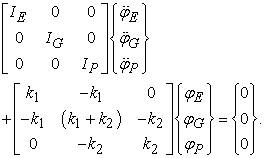(1)

For free vibrations, which have the single harmonic motion (SHM), it takes the form:(2)

Where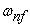is the torsional natural frequency of the system. Natural frequencies can be obtained by obtaining characteristic equations (or frequencies). For this purpose, we put the matrix in equation (2) to zero and we obtain the characteristic equation as follows:(3)

Solving this equation, the natural frequencies of the system are found as follows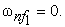(4)

And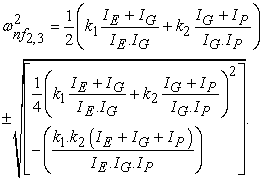(5)

The torsional stiffness of the shaft,is equal to the applied torque divided by the angle of twist.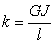(6)

wheremodulus of rigidity for shaft material,second moment of area about the axis of rotation and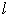length of shaft.

### 3. Results

Whirling can cause damage such as fatigue, wear of the stern tube bearing and oil leaks in sealing and causing other problems, such as a damage of the propeller shaft, stern tube bearings and stern tube sealing device. In particular, in some cases, whirling can sustain the local vibration of the body in the stern of the ship.

For this purpose, three examples of the propulsion system have been studied and analyzed. In these examples, the speeds of whirling, which is the same as the natural frequency of vibration, are obtained, and the effect of the torsional stiffness and shaft diameter have been investigated.

Example 1:

First example is shown in Figure 4 that is 4DOF system

• Figure 4. Single branched torsional system

Data are given as follows: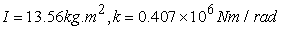Gear ratio =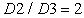.

The equivalent system for the problem can be represented in Figure 5.

• Figure 5. Equivalent system for single branched torsional system for Figure 4

Mass matrix,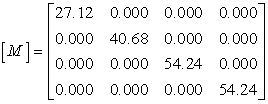(7)

Stiffness matrix,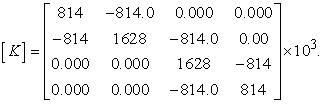(8)

For results we need to solve the eigenvalue problem defined as,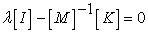(9)

whereandis unit matrix.

So, all 4 natural frequencies are found.As shown in Table 1, the results obtained from the analytical method are very good compliance with the results of finite element method 16.

The effect of shaft diameter and torsional stiffness of the shaft on the whirling speed is presented on Table 2 and Table 3 and also shown in Figure 6.

As previously described, we increase the amount of the diameter and stiffness of the shaft in four steps and each step by five percent. The values obtained for whirling speed are higher due to the shaft diameter increase relative to the values obtained for the whirling speed due to torsional stiffness of the shaft, and this difference is more than the higher frequencies. Table 4 is given the whirling speed in RPM at different diameters and different torsional stiffness.

• Figure 6. Whirling speeds at various shaft diameter and torsional stiffness (example 1)

Figure 6 shows that variations in the whirling speed at higher natural frequencies are due to increased diameter and torsional stiffness of the shaft.

Example 2:

For a geared system as shown in Figure 7, we find torsional natural frequencies. The shaft ‘A’ has 5 cm diameter and 0.75 m length, and the shaft ‘B’ has 4 cm diameter and 1.0 m length. Take the modulus of rigidity of the shaft G equals tothe polar mass moment of inertia of discs and gears are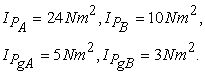• Figure 7. Two-discs with a geared system

The torsional stiffness of shaft segments (1) and (2) are given as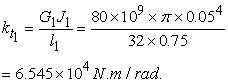And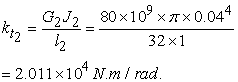Table 5, Table 6 and Table 7 and also Figure 8 are shown the results of the whiling speeds at various diameters and torsional stiffness.

• Figure 8. Whirling speeds at various shaft diameter and torsional stiffness (example 2)
Example 3:

A marine reciprocating engine, flywheel and propeller are approximately equivalent to the following three-rotor system (Figure 9). The engine has a crank 50 cm long and a connecting rod 250 cm long. The engine revolving parts masses are equivalent to 96.59 kg at crank radius. The reciprocating mass is 46.41 kg. The mass of the flywheel is 200 kg with the radius of gyration of 25 cm. The propeller has the polar mass moment of inertia of 6 kg-m2. The equivalent shaft between the engine masses and the flywheel is 38 cm diameter and 5.3 m long and that between the flywheel and the propeller is 36 cm diameter and 1.5 m long. Find the natural frequencies of the torsional vibrations of the system.

Solution: The main aim of the present solution procedure would be to first find the equivalent rotating mass of the reciprocating engine, once it has been done then the problem will reduce to obtaining the natural frequencies of a three-rotor system as shown in Figure 8. The three revolving masses are corresponding to the reciprocating engine, flywheel, and propeller.

• Figure 9. A three-disc model of the engine, flywheel and propeller

Hence, the equivalent polar mass moment of inertia of the engine is obtained as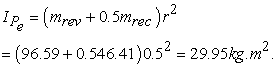Now, the polar mass moment of inertia of the flywheelFor the propeller, the polar mass moment of inertia is given as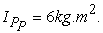The torsional stiffness of shaft segments (1) and (2) are given asAnd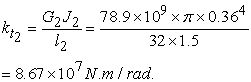The results of the whirling speeds are given in Table 8, Table 9 and Table 10 and also Figure 10 at various diameters and stiffness.

• Figure 10. Whirling speeds at various shaft diameter and torsional stiffness (example 3)

As shown in Figure 6, Figure 8 and Figure 10, due to increase in the diameter and torsional stiffness of the shaft in propulsion shafting systems with and without gearboxes, the rate of variation of the whirling speed is found at higher frequencies, while it does not change at low frequencies.

### 4. Conclusions

Whirling speed is obtained for various shafting system obtained for three examples. The effect of shaft diameter and torsional stiffness are presented and discussed. The amount of critical speed variation due to the increased in shaft diameter is more than the critical speed variation due to the increased torsional stiffness of the shaft. In all three examples, by increasing diameter and torsional stiffness of the propeller shaft, the natural frequency of the propeller shaft increases.

### References

  Yang, S.M., Sheu, G.R., Yang, C.D., “Analytical Solution of Whirl Speed and Mode Shape for Rotating Shafts”, American Society of Mechanical Engineers, 1998. In article View Article  Suk Sun, J., Min Han, T., Ki Lee, K., Kan Kim, U., “A Study on the Measurement and Analysis of Whirling Vibration Behavior of Marine Propulsion Shafting System using Gap-sensors”, Journal of the Korean Society of Marine Engineering, 39(2), pp 130-135, 2015. In article View Article  Batrak, Y., Batrak, R., Berin, D., Mikhno, A., “Propulsion shafting whirling vibration: case studies and perspectives”, Intellectual Maritime Technologies, Mykolaiv, Ukraine, 2015. In article  Ku, M., “Finite element analysis of whirl speeds for rotor-bearing systems with internal damping”, Mechanical Systems and Signal Processing, Vol. 12, pp 599-610, 1998. In article View Article  Shahgholi, M., Khadem, S.E., “Internal, combinational and sub-harmonic resonances of a nonlinear asymmetrical rotating shaft”, Springer Science and Business Media Dordrecht, 2014. In article PubMed  Shahgholi, M., Khadem, S.E., “Primary and parametric resonances of asymmetrical rotating shafts with stretching nonlinearity”, Springer Mechanism and Machine Theory, Vol. 51, pp 131-144, 2012. In article View Article  Tailony, Ra., “New Modeling Approach for Engine Cold Testing Driveline Torsional Vibration Analysis with Increased Accuracy”, American Journal of Mechanical Engineering, 5(4), pp 156-160, 2017. In article View Article  Wu, J.Sh. Lin, F.T., Shaw, H.J., “Analytical solution for whirling speeds and mode shapes of a distributed-mass shaft with arbitrary rigid disks”, ASME, Vol. 81, pp 1-10, 2014. In article PubMed  Desavale, R.G., Patil, A.M., “Theoretical and Experimental Analysis of Torsional and Bending Effect on Four Cylinders Engine Crankshafts by Using Finite Element Approach”, International Journal of Engineering Research, 2(6), pp 379-385, 2013. In article View Article  Zou, D., Jiao, Ch., Ta, Na., Rao, Zh. “Forced vibrations of a marine propulsion shafting with geometrical nonlinearity (primary and internal resonances)”, Mechanism and Machine Theory, Vol. 105, pp 304-319, 2016. In article View Article  Zou, D., Liu, L., Rao, Zh. Ta, Na., “Coupled longitudinal–transverse dynamics of a marine propulsion shafting under primary and internal resonances”, Journal of Sound and Vibration, Vol. 372, pp 299-316, 2016. In article View Article  Tecnavin, S.A., “Analysis of most frequent cases of vibration in propulsion systems”, Ship Science & Technology, Vol. 7, pp 65-74, 2013. In article View Article  Luczko, J., “A geometrically non-linear model of rotating shafts with internal resonance and self-excited vibration”, Sound and vibration, 255(3), pp 433-456, 2002. In article View Article  Murthy BSN, Srinivas J., Singh R.P., Sri K.U., Kiran L., “Dynamic Analysis of a Dual-Disk Rotor Bearing System with Parametric Excitations”, Journal of Mechanical Design and Vibration, 2014, 2(4), pp81-86. In article View Article  Beards, C.F., “Engineering Vibration Analysis with Application to Control Systems”, Butterworth-Heinemann 1995. In article View Article  Kushwaha, V., “Analysis of torsional vibration characteristics for multi-rotor and gear-branched systems using finite element method”, National Institute of Technology Rourkela, chapter 4, pp 39-41, 2011-2012. In article View ArticleThis work is licensed under a Creative Commons Attribution 4.0 International License. To view a copy of this license, visit http://creativecommons.org/licenses/by/4.0/